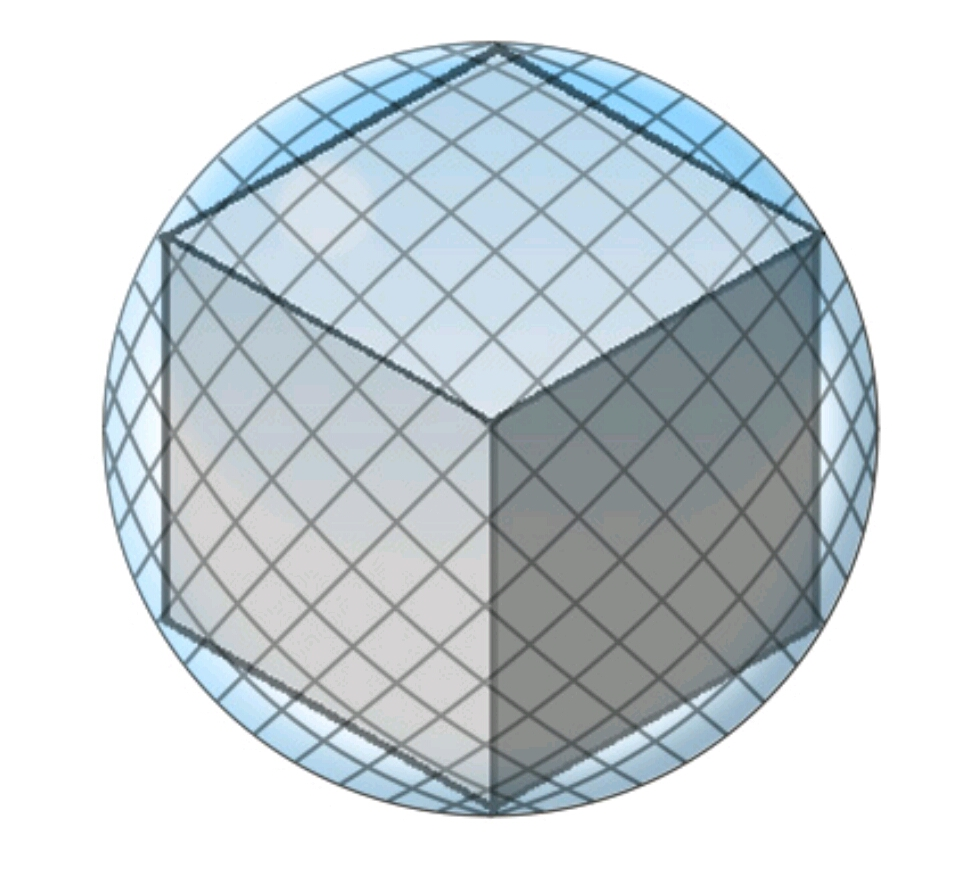# Sphere pressureA cube rests inside a sphere such that each vertex touches the sphere. The radius of the sphere is $6 \text{ cm}.$ Determine the volume of the cube.

If the volume of the cube can be expressed in the form of $a\sqrt{3} \text{ cm}^{3}$, find the value of $a$.

×